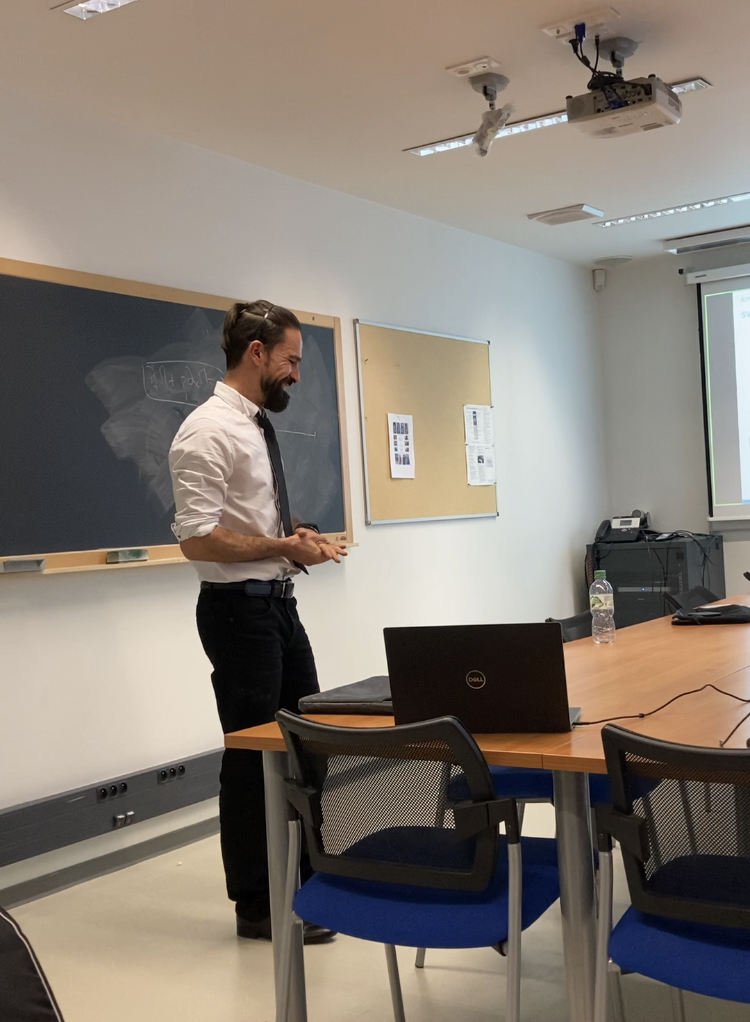# Laboratoire IMATH

Institut de Mathématiques de Toulon (EA 2134)

## Soutenance HDR de Mehmet ERSOY (IMATH)

Soutenance HDR MN
Mardi 01/12/20, 14h, salle M141

From hydrostatic to non-hydrostatic models in fluid mechanics : modeling, mathematical and numerical analysis, and computational fluid dynamics.

Abstract : This dissertation is the fruit of my last ten years of research at IMATH. It concerns the mathematical, numerical analysis of compressible and incompressible equations in which I tackle hydrostatic and non- hydrostatic equations.
I present and develop numerical tools for adaptive mesh refine- ment on unstructured meshes in any dimension for finite volume and discontinuous Galerkin methods.
I also propose model reduction, i.e. asymptotic approximation, in the long wave framework to derive or revisit hydrostatic and non- hydrostatic nonlinear models. In particular, I propose the first gen- eralisation of the one-dimensional Serre-Green-Naghdi equations for arbitrary geometry for river hydraulics. This is achieved by a 3D to 1D reduction.